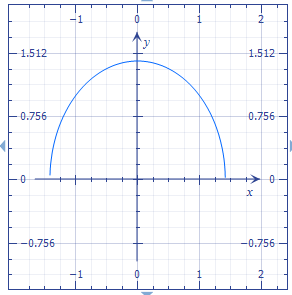# Draw a rough picture of the following surface and also clarify some basic geometric properties...

## Question:

Draw a rough picture of the following surface and also clarify some basic geometric properties (e.g. axis, vertex): {eq}x^2 + z^2 = 4 {/eq}.

## Graph the functions

We can graph a function in the Cartesian plane or coordinate system when the function is of a variable. The behavior can be seen in the graph, we can also see the geometric properties.

We have, {eq}x^2 + z^2 = 4 \\ z^2=4-x^{2} \\ z=f(x)= \sqrt{ 2-x^{2} } \\ {/eq}

Graph the function {eq}f(x)= \sqrt{ 2-x^{2} } \\ {/eq}The function has the geometric shape of a semicircle